## Quiz 7 : Rate-Of-Return AnalysisLooking for Economics Homework Help?# Quiz 7 : Rate-Of-Return Analysis

Interest rate : It is periodic percentage apply to a particular amount in order to estimate the interest earned on that sum. Consider one invest \$1,000 in stock and is considered a financial asset, a two year later, the same investment yields \$1,864, then the rate of return of the given situation is calculated as follows. To find the unknown interest rate use the following formula: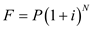Where, The future amount of the lump sum of money be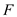. The present value of the sum of money be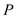. The interest rate be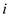. The number of terms or period be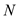. Substitute the given values in the above equation and solve as follows: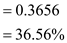Hence, the return on the investment over two years of period is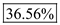.

The seventh chapter of the textbook focuses on various ways that a person can determine what the return on an investment may be for a given situation. Some of the topics mentioned include ways to determine interest rates, accept, or reject decisions, and more. The problem of focus here in the text is a short case study that focuses on technologies that one can use to pay for purchases at a store. These include an option that takes fingerprints for payment or a card that does not have to be swiped and is waved in front of an infrared reader. As one goes deeper into the problem, it is clear that the Pay by Touch TM system is the one that is more appealing and details are provided which shows costs and the like for the scanning equipment. Assuming that one is an owner of 28,000 stores in the nation, how much additional revenue would it take to use this system Note that one is looking for a rate of return of 20.00% at the minimum. While the problem doesn't look too bad on the surface, there are a couple of things that we will need to do here in order to get a ballpark idea as to what the amount of revenue will be needed above and beyond operating and maintenance costs for the machine in question. To assist with this, here is a summary list of details and assumptions that can be made for this problem with respect to the new transaction system: The initial cost of the machine is \$50.00; Monthly subscription fees for each store unit per an agreement is \$40.00; It can be assumed that each store will have about 1,000 transactions a month; o Note that some stores will be higher and some will be lower naturally. It is guestimated that the product will have a useful life of five (5) years; There is no salvage value on the unit as it will likely be wore out well before then due to heavy use; and The required minimum attractive rate of return is 20.00% per year (or an effective rate of 1.53% per month as we are calculating it here). Now that we have the details, all we need to do is to find the present worth for a monthly period when using this new technology for the stores one owns. This is shown below as the calculations applicable to the situation: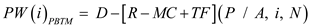…… (1) Where… - PW(i) = the present worth (also known as NPW or P ), - P = present value of the sum of money, - A = annual costs for the situation at hand, - D = the initial cash flow in the series focused on here, - R = the revenue required in order to satisfy the equipment at some level, - MC = the maintenance costs for one (1) period of time, - TF = the transaction fees (based on customer volume mentioned), - i = interest rate (which is known in this case), and - n = number of terms that the money is for. With the equation in hand, let us solve for the net present worth so that we can see what the present worth is going to be for the option reviewed. Shown below to help save space and a bit of time are Alternative A and the way that one would calculation the cash flow series here:The next thing that should be done here is to mosey on over to the back of the textbook to get the values for the factor that is shown above. Note that the factor applicable here will be in the fifth column for the factor applicable here. However, we do have a bit of a problem here. Once can scour through the tables for a few minutes to try to find an educated guess as to where it may land but using an online calculator is a better option to get the required answer here. This will be a helpful tool for not only this problem for many others as well similar to this. The factor value applicable here are approximately 39.0753 and we continue below:When we see the number above, what that is saving that it will take \$140.51 of revenue to run the machine for one month. It is very interesting that the amount to run a machine like this is quite low in order for this to be paid off so to speak in terms of cost. While most people may fret about the technology, at least in this assumption and customer volume, it is actually a manageable cost.

It is given that the amount of car that needs to be financed is \$24,500. The borrower is required to pay \$514.55 for 60 months. Following is the formula to calculate the rate of return: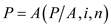Here, P is the amount of loan (\$24,500) A is the amount of uniform payment (\$514.55) i is the rate of return (= ) n is the time period (60 months) Substitute the values in above formula.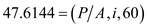…… (1) Look through the compound interest table and apply trial and error method and find an interest rate which equalizes equation (1). That is, find an interest rate which equalizes left-hand side and right-hand side of equation (1). Substituting the tabular values into the right-hand side of equation (1). The table below shows the two options whose values are closer to left-hand side of equation (1).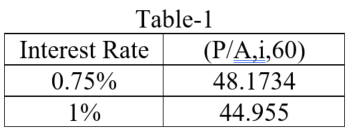The rate of return, which is between 0.75% and 1%, may indeed be computed by linear interpolation.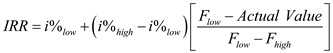…… (2) Here,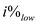= lower interest rate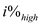= higher interest rate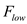= factor of lower interest rate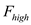= factor of higher interest rate Substituting the values in equation (2), the result is as follows: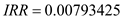Therefore, the monthly rate of return is 0.793425% (approx.) Following is the formula to calculate annual nominal rate of interest (assuming there are 12 months in year):Thus, the annual nominal rate of interest is 9.5211% Following is the formula to calculate effective interest rate: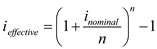Here, i nominal is the nominal annual interest rate (9.5211% or 0.095211) n is the number of compounding period in year (12 months) Substitute the values in above equation.Thus, the effective annual interest rate is 9.95%